Processing ......FreeComputerBooks.com Links to Free Computer, Mathematics, Technical Books all over the World

Theory of the Integral
Top Free Web Programming Books 🌠 - 100% Free or Open Source!
• Title Theory of the Integral
• Author(s) Brian S. Thomson
• Publisher: CreateSpace (February 10, 2013); eBook (Update Continuously)
• Paperback 422 pages
• eBook PDF
• Language: English
• ISBN-10: 1467924393
• ISBN-13: 978-1467924399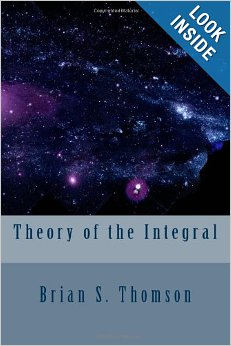Book Description

This text is intended as a treatise for a rigorous course introducing the elements of integration theory on the real line. All of the important features of the Riemann integral, the Lebesgue integral, and the Henstock-Kurzweil integral are covered.

The text can be considered a sequel to the four chapters of the more elementary text The Calculus Integral which can be downloaded from our web site. For advanced readers, however, the text is self-contained.

• Brian S. Thomson is a Professor Emeritus of Mathematics, Simon Fraser University
Reviews, Ratings, and Recommendations: Related Book Categories: Read and Download Links:Similar Books:
•Measure, Integration and Real Analysis (Sheldon Axler)

This textbook welcomes students into the fundamental theory of measure, integration, and real analysis. Focusing on an accessible approach, it lays the foundations for further study by promoting a deep understanding of key results.

•Complex Integration and Cauchy's Theorem (G.N. Watson)

This brief monograph by one of the great mathematicians offers a single-volume compilation of propositions employed in proofs of Cauchy's theorem, provides a brief account of the various applications of the theorem to the evaluation of definite integrals.

•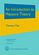An Introduction to Measure Theory (Terrence Tao)

This is a graduate text introducing the fundamentals of measure theory and integration theory, which is the foundation of modern real analysis, intended to cover a quarter or semester's worth of material for a first graduate course in real analysis.

•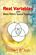Real Variables with Basic Metric Space Topology (Robert B. Ash)

Designed for a first course in real variables, this text presents the fundamentals for more advanced mathematical work, particularly in the areas of complex variables, measure theory, differential equations, functional analysis, and probability.

•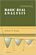Basic Real Analysis (Anthony W. Knapp)

This is a comprehensive treatment with a global view of the Real Analysis, emphasizing the connections between real analysis and other branches of mathematics. Included throughout are many examples and hundreds of problems.

•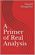A Primer of Real Analysis (Dan Sloughter)

This is a short introduction to the fundamentals of real analysis, written the text assuming the reader has the level of mathematical maturity of one who has completed the standard sequence of calculus courses.

•Calculus (Gilbert Strang)

This highly regarded calculus textbook is ideal as for self-study. Examples of apps of calculus to physics, engineering and economics are included, as well as many practice questions and illustrative diagrams to assist the reader's grasp of the material.

•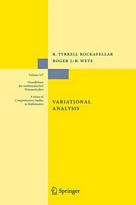Variational Analysis (R. Tyrrell Rockafellar, et al)

This book develops a unified framework and, in finite dimension, provides a detailed exposition of variational geometry and subdifferential calculus in their current forms beyond classical and convex analysis.

Book Categories
 :All CategoriesTop Free BooksRecent BooksMiscellaneous BooksComputer EngineeringComputer LanguagesComputer ScienceData Science/DatabasesJava and Java EE (J2EE)Linux and UnixMathematicsMicrosoft and .NETMobile ComputingNetworking and CommunicationsSoftware EngineeringSpecial TopicsWeb Programming
Other Categories